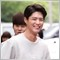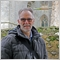116

Hi, experienced coders out there , please i need some help in adding alert sound when the 1 2 3 is printed on my chart , thank you for your kindness!!

Here is the code ..

#property indicator_buffers 2
#property indicator_color1 Red
#property indicator_color2 Lime

extern int  maxbars=200;
extern int aggression=1;
extern string fontname="Arial Black";
extern int fontsize=8;
extern int PipTextHeight=0;

int oldmaxbars=0;
double estpiptexth=0.0;
int lastDNcheckpos=0;
int lastUPcheckpos=0;

//+------------------------------------------------------------------+
//| Custom indicator initialization function                         |
//+------------------------------------------------------------------+
int init()
{
//---- indicators
//----

return(0);
}

void movemarkers(string obname)
{
double yp=0;
int obindx=0;
string tstr;
int pos;

pos=0;
while (Time[pos] > StrToDouble(StringSubstr(obname,7,StringLen(obname)-7))) pos++;

if ((StringSubstr(obname,3,3) ==  "Dp2") || (StringSubstr(obname,3,3) ==  "Up1") || (StringSubstr(obname,3,3) ==  "Up3"))
{
/// move markers in bottom pos
yp = Low[pos];
tstr ="123Dp2,"+StringSubstr(obname,7,StringLen(obname)-7);
obindx = ObjectFind(tstr);
if (obindx !=-1)
{
ObjectSet(tstr,OBJPROP_PRICE1,yp);
yp=yp-estpiptexth;
}

tstr ="123Up1,"+StringSubstr(obname,7,StringLen(obname)-7);
obindx = ObjectFind(tstr);
if (obindx !=-1)
{
ObjectSet(tstr,OBJPROP_PRICE1,yp);
yp=yp-estpiptexth;
}

tstr ="123Up3,"+StringSubstr(obname,7,StringLen(obname)-7);
obindx = ObjectFind(tstr);
if (obindx !=-1)
{
ObjectSet(tstr,OBJPROP_PRICE1,yp);
yp=yp-estpiptexth;
}

}
else
{
//move markers in top pos
yp = High[pos]+estpiptexth;
tstr ="123Up2,"+StringSubstr(obname,7,StringLen(obname)-7);
obindx = ObjectFind(tstr);
if (obindx !=-1)
{
ObjectSet(tstr,OBJPROP_PRICE1,yp);
yp=yp+estpiptexth;
}

tstr ="123Dp1,"+StringSubstr(obname,7,StringLen(obname)-7);
obindx = ObjectFind(tstr);
if (obindx !=-1)
{
ObjectSet(tstr,OBJPROP_PRICE1,yp);
yp=yp+estpiptexth;
}

tstr ="123Dp3,"+StringSubstr(obname,7,StringLen(obname)-7);
obindx = ObjectFind(tstr);
if (obindx !=-1)
{
ObjectSet(tstr,OBJPROP_PRICE1,yp);
yp=yp+estpiptexth;
}

}
}

void deleteoldmarkers(string mtype,int frompos)
{
int pos=0;
string tstr;

// delete old 123 text markers
// if frompos = -1 then delete all markers
// els delete markers younger than the date supplied

pos = 0;
while (pos < ObjectsTotal())
{
tstr = ObjectName(pos);
if (StringSubstr(tstr,0,3+StringLen(mtype)) =="123"+mtype)
{
if (frompos ==-1)
{
ObjectDelete(tstr);
pos = 0;
}
else
{
if (StrToDouble(StringSubstr(tstr,7,StringLen(tstr)-7)) >= frompos)
{
ObjectDelete(tstr);
//move any other markers at this point.
movemarkers(tstr);
pos = 0;
}
else pos++;
}
}
else
pos++;
}
}

//+------------------------------------------------------------------+
//| Custom indicator deinitialization function                       |
//+------------------------------------------------------------------+
int deinit()
{

deleteoldmarkers("",-1);
return(0);
}

bool obinset(string obname,string typeset)
{

if (typeset=="T")
{
// check if we have one 1 on top of price bar
if ((StringSubstr(obname,0,6) =="123Dp1") || (StringSubstr(obname,0,6) =="123Dp3") ||
(StringSubstr(obname,0,6) =="123Up2")) return(true);
}
else
if (typeset=="B")
{
// check if we have one 1 on bottom of price bar
if ((StringSubstr(obname,0,6) =="123Up1") || (StringSubstr(obname,0,6) =="123Up3") ||
(StringSubstr(obname,0,6) =="123Dp2")) return(true);
}
return(false);
}

int findobatpos(int barnum,string pointtype,string typeset)
{
int inx=0;
int res=0;
string tstr;
string fstr;

// count  markers at datetime supplied in barnum
/// if point type = "" then count any 123 marker type
/// else only count markers of type detailed in pointtype
/// return number of markers at Point
inx = ObjectsTotal();
while ((inx >=0)&& (res==0))
{
tstr = ObjectName(inx);
fstr = "123"+pointtype;
if (((StringSubstr(tstr,0,StringLen(fstr)) ==fstr) && (typeset =="")) ||
((typeset!= "") && (obinset(tstr,typeset)) ))
{
if (StrToDouble(StringSubstr(tstr,7,StringLen(tstr)-7)) == barnum)
{
res++;
inx--;
if (pointtype =="")
{
while (inx >=0)
{
tstr = ObjectName(inx);
fstr = "123"+pointtype;
if (((StringSubstr(tstr,0,StringLen(fstr)) ==fstr) && (typeset=="")) ||
((typeset!= "") && (obinset(tstr,typeset)) ))
{
if (StrToDouble(StringSubstr(tstr,7,StringLen(tstr)-7)) == barnum)
res++;
}
inx--;
}
}

}
}
inx--;
}

return(res);
}

double getestpiptexth()
{
double range=0;
int st=0;
double result;
int texth=0;

/// cacluate & return the estimated text height in pips

st=FirstVisibleBar();

st = st-BarsPerWindow();

if (st <0) st=0;

range =High[Highest(NULL,0,MODE_HIGH,BarsPerWindow(),st)];

range = range - Low[Lowest(NULL,0,MODE_LOW,BarsPerWindow(),st)];

// add 8% of range to estimate window height in pips
range = range+((range*0.08)*Point);

// scale the fontsize use result var cos we need a double not int
result = fontsize;
texth= MathRound((result /8)*4);

result = (Point*texth)*(range/(range/(range/(Point*100))));

if (result < Point ) result = Point;

return(result);

}

void CheckDowns()
{
int pos=0;
int dn1pos =-1;
int dn2pos=-1;
int dn3pos=-1;

//check for down 123s

pos = lastDNcheckpos;

while ((pos >0) && (!IsStopped() ))
{

// get to bottom of down run if we are in one
while ((pos >0) && (High[pos+1]>= High[pos])) pos--;

/// find potential 1 & 2
while ((pos >0) && (dn1pos ==-1))
{
if (!(High[pos+1]<= High[pos]))
{
dn1pos =pos+1;
dn2pos = pos;
}
else pos--;
}

pos--;

//if we dont have a pos 3 then find one or invalidate pos1&2
while ((pos>0) && (dn3pos==-1))
{

if (High[pos] >High[dn1pos])
{
pos = dn1pos-1;
dn1pos =-1;
dn2pos =-1;
dn3pos=-1;
break;
}
else
if (Low[pos]< Low[dn2pos])
{
dn2pos = pos;
pos--;
}
else
if (High[pos] > High[dn2pos])
{
dn3pos = pos;
}
else pos--;
}

// we have found a potential 3 so check if we have a higher bar
if (dn3pos !=-1)
{

pos--;
if (pos==0)
{
if (High > High[dn1pos]) dn3pos =-1;
}
else
{

//done = false;
while ((pos >0)   && (dn3pos !=-1))
{
if (High[pos] > High[dn1pos])
{
pos = dn1pos-1;
dn1pos =-1;
dn2pos=-1;
dn3pos=-1;
}
else
if ((Low[pos] < Low[dn3pos])&& (High[pos]<= High[dn3pos]))
{
break;
}
else
if (High[pos] >= High[dn3pos])
{
dn3pos = pos;
pos--;
}
else pos--;
}

}
}

if ((dn1pos !=-1) && (dn2pos !=-1) && (dn3pos !=-1) )
{

if ((findobatpos(Time[dn1pos],"Dp3","") > 0) && (aggression <3))
{
if (aggression < 2 ) pos = dn2pos-1; else pos=dn1pos-1;
dn1pos =-1;
dn2pos=-1;
dn3pos=-1;
}
else
if  ((findobatpos(Time[dn3pos],"Dp3","") > 0) && (aggression == 0))
{
if (aggression < 2 ) pos = dn2pos-1; else pos=dn1pos-1;
dn1pos =-1;
dn2pos=-1;
dn3pos=-1;
}
else
{
if  (!IsStopped())
{

ObjectSetText("123Dp1,"+DoubleToStr(Time[dn1pos],0),"1",fontsize,fontname,indicator_color1);
}

if  (!IsStopped())
{
ObjectSetText("123Dp2,"+DoubleToStr(Time[dn2pos],0),"2",fontsize,fontname,indicator_color1);
}

if  (!IsStopped())
{
ObjectSetText("123Dp3,"+DoubleToStr(Time[dn3pos],0),"3",fontsize,fontname,indicator_color1);
}
lastDNcheckpos = Time[dn1pos];
if (aggression < 2) pos = dn2pos-1; else pos=dn1pos-1;
dn1pos =-1;
dn2pos=-1;
dn3pos=-1;
}
}
}
}

void CheckUps()
{
int pos=0;
int up1pos =-1;
int up2pos=-1;
int up3pos=-1;

//check for Up 123s

pos = lastUPcheckpos;

while ((pos >0) &&(!IsStopped()))
{

// get to top of up run if we are in one
while ((pos >0) && (Low[pos+1]<= Low[pos])) pos--;

/// find potential 1 & 2
while ((pos >0) && (up1pos ==-1))
{
if (!(Low[pos+1]>= Low[pos]))
{
up1pos =pos+1;
up2pos = pos;
}
else pos--;
}

pos--;

//if we dont have a pos 3 then find one or invalidate pos1&2
while ((pos>0) && (up3pos==-1))
{

if (Low[pos] <Low[up1pos])
{
pos = up1pos-1;
up1pos =-1;
up2pos =-1;
up3pos=-1;
break;
}
else
if (High[pos]> High[up2pos])
{
up2pos = pos;
pos--;
}
else
if (Low[pos] < Low[up2pos])
{
up3pos = pos;
}
else pos--;

}

// we have found a potential 3 so check if we have a higher bar
if (up3pos !=-1)
{

pos--;
if (pos==0)
{
if (Low < Low[up1pos]) up3pos =-1;
}
else
{

while ((pos >0) && (up3pos !=-1))
{
if (Low[pos] < Low[up1pos])
{
pos = up1pos-1;
up1pos =-1;
up2pos=-1;
up3pos=-1;
}
else
if ((High[pos] > High[up3pos])&& (Low[pos]>= Low[up3pos]))
{
break;
}
else
if (Low[pos] <= Low[up3pos])
{
up3pos = pos;
pos--;
}
else
pos--;
}

}
}

if ((up1pos !=-1) && (up2pos !=-1) && (up3pos !=-1))
{
if ((findobatpos(Time[up1pos],"Up3","") > 0) && (aggression <3))
{
if (aggression < 2) pos = up2pos-1; else pos=up1pos-1;
up1pos =-1;
up2pos=-1;
up3pos=-1;
}
else
if   ((findobatpos(Time[up3pos],"Up3","") > 0)&& (aggression ==0))
{
if (aggression < 2) pos = up2pos-1; else pos=up1pos-1;
up1pos =-1;
up2pos=-1;
up3pos=-1;
}
else
{
if  (!IsStopped())
{
ObjectSetText("123Up1,"+DoubleToStr(Time[up1pos],0),"1",fontsize,fontname,indicator_color2);
}

if  (!IsStopped())
{
ObjectSetText("123Up2,"+DoubleToStr(Time[up2pos],0),"2",fontsize,fontname,indicator_color2);
}

if  (!IsStopped())
{
ObjectSetText("123Up3,"+DoubleToStr(Time[up3pos],0),"3",fontsize,fontname,indicator_color2);
}
lastUPcheckpos = Time[up1pos];
if (aggression < 2) pos = up2pos-1; else pos=up1pos-1;
up1pos =-1;
up2pos=-1;
up3pos=-1;
}
}
}
}

int start()
{
int tpos=0;
double tmpeh;
string tstr="";

if (IsStopped()) return(0);

// if user changed maxbars then force reset of all
if (maxbars !=oldmaxbars)
{
oldmaxbars = maxbars;
if (maxbars > Bars )
{
maxbars =Bars;
oldmaxbars =maxbars;
}
estpiptexth =0.0;
}

// see if everything needs to be recalced
if (PipTextHeight == 0)
{
//if user has not specified a piptext height then
tmpeh =getestpiptexth();
if (tmpeh != estpiptexth)
{
estpiptexth = tmpeh;
lastDNcheckpos = maxbars;
lastUPcheckpos = maxbars;
deleteoldmarkers("",-1);
}

}
else
{

//if user has specified a piptext height then
if (estpiptexth != (PipTextHeight *Point))
{
estpiptexth = (PipTextHeight *Point);
lastDNcheckpos = maxbars;
lastUPcheckpos = maxbars;
deleteoldmarkers("",-1);
}
}

if (lastUPcheckpos != maxbars)
{
lastUPcheckpos =0;

while ((lastUPcheckpos < maxbars) && (findobatpos(Time[lastUPcheckpos],"Up1","") == 0)) lastUPcheckpos++;
while ((lastUPcheckpos < maxbars) && (findobatpos(Time[lastUPcheckpos],"Up3","") == 0)) lastUPcheckpos++;
tpos = lastUPcheckpos+1;
lastUPcheckpos--;

if (aggression <2)  tstr = "Up2"; else tstr= "Up1";

while ((tpos < maxbars) && (findobatpos(Time[tpos],tstr,"") == 0)) tpos++;

deleteoldmarkers("U",Time[lastUPcheckpos]);
lastUPcheckpos = tpos-1;
}

if (lastDNcheckpos != maxbars)
{
lastDNcheckpos =0;

while ((lastDNcheckpos < maxbars) && (findobatpos(Time[lastDNcheckpos],"Dp1","") == 0)) lastDNcheckpos++;
while ((lastDNcheckpos < maxbars) && (findobatpos(Time[lastDNcheckpos],"Dp3","") == 0)) lastDNcheckpos++;
tpos = lastDNcheckpos+1;
lastDNcheckpos--;

if (aggression <2)  tstr = "Dp2"; else tstr= "Dp1";

while ((tpos < maxbars) && (findobatpos(Time[tpos],tstr,"") == 0)) tpos++;

deleteoldmarkers("D",Time[lastDNcheckpos]);
lastDNcheckpos = tpos-1;
}

if (!IsStopped())
{
CheckDowns();
CheckUps();
}

RefreshRates();
return(0);
}
//+------------------------------------------------------------------+
Files:
sshot-6.png 27 kb167

Hello,

Is it possible to check the Alert option in MQL5? I guess this option is turned off in your MQL5.

In MQL5 Press Ctr+O and select the tab "Events".